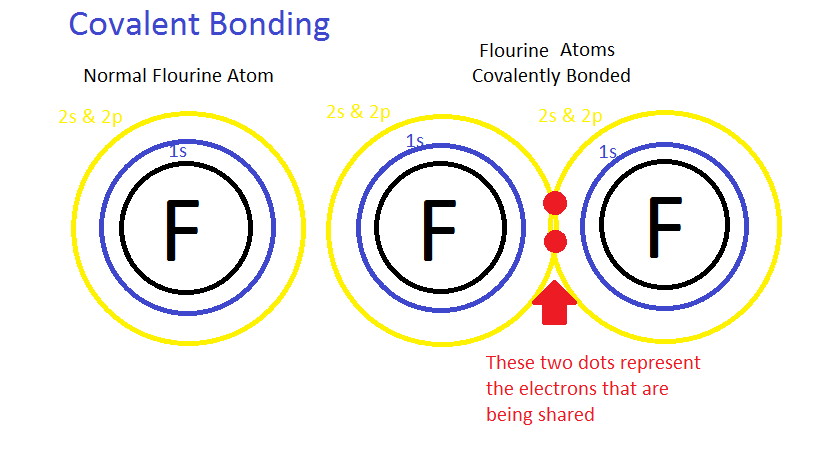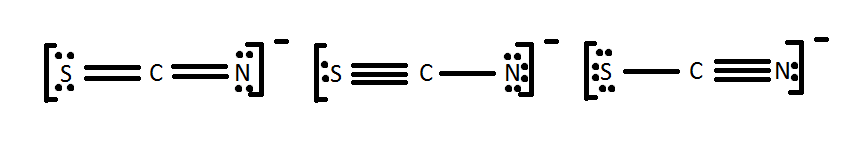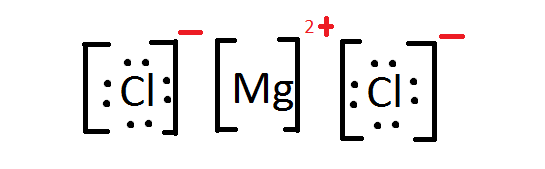1.2: Lewis Theory of Bonding

Chemical bonding is at the heart of understanding chemistry. The Lewis Theory of Bonding essentially combined observations at the time about chemical bonding together. Not only was it essential in understanding how elements bonded, it provided a visual representation for them. These Lewis dot structures are a simplistic way of representing the electrons in molecules. This theory also helped to define formal charge and resonance. Understanding of electronegativity and electron affinity will be useful before understanding Lewis Theory of Bonding.

Introduction

The Lewis Theory used observations from chemists and physicists to form a theory about chemical bonding. This work was essentially a compilation of the knowledge at the time. It revolved around the importance of valence electrons in chemical bonding. These are the electrons that are in the outermost shell. For example Na may have 11 electrons, but only one is a valence electron, the one in 3s1. Meanwhile P has 15 electrons, but has five valence electrons, 3s2 and 3p2. The bonding of an element is based on how they fill their octets i.e. achieve a noble gas electron configurations.

Lewis went on to explain how certain elements such as Boron did not necessarily follow these same rules. He explicitly defined two ways electrons were used to form bonds. These were ionic (complete transfer of electron) and covalent (sharing of electrons) bonding. He added to his covalent bonding theory that atoms can have a double or triple bonds with other atoms when electrons are shared. A single bond consists of two electrons sharing between two atoms, double bond consists of four electrons sharing between two atoms, and triple bond consists of six electrons sharing between two atoms. In both cases Lewis dot structures were used to visualize the the bonding of the atoms in case of electron sharing or electron transfer.

Basic Lewis Dot Structures

There are two basic types of bonding that form the basis of the Lewis theory and it is important to understood before one can begin to draw Lewis dot structures.

Ionic Bonding

In ionic bonding one element is much more electronegative then another element. In other words, the electronegative atom wants an electron much more than the other element; thus, the element with a positive charge loses electron and the element with the negative charge gains an electron. These two elements then become attracted to each other because of Coulomb’s Law which states that there is electrostatic interaction between particles that are electrically charged, in this case the electrons describes the charge of the atom. The atom that loses electrons is electron deficient; thus, the atomic charge will be positive. The atom that gains electrons has excess of electrons; thus, the atomic charge will be negative. This attraction between the elements brings them together to form an ionic bond. An example of an ionic compound is shown below.Covalent Bonding

In covalent bonding the elements share electrons equally, i.e. both the atoms have an equal share of electrons in the bond. This sharing of electrons creates a bond that is known as a covalent bond. It is thought that a covalent bond is equal, but in nature, the bond is never equal. The electronegativity differs between all the elements and the more electronegative the atom, it has a higher tendency to gain electrons; however, because the bond is formed by sharing electrons, the atom cannot "possess" an electron. So, the only way for the more electronegative atom to take the "advantage" of an electron is by unequal sharing of electrons. The compounds that share electrons unequally are called polar compounds and the process is called polarity. An example of a polar compound is water (H2O). An example of a generalized covalent compound is shown below.How to Draw Lewis Structure: To begin any Lewis dot structure, begin by counting how many valence electrons are in all the elements.

CH4 CO2

1 C: 1 * [4 Valence Electrons] (2s22p2) 1 C: 1 * [4 Valence Electrons] (2s22p2)

4 H: 4 * [1 Valence Electron] (1s1) 2 O: 2 * [6 Valence Electrons] (2s22p4)

4+4 = 8 Valence Electrons 4 + 12 = 16 Valence Electrons

Next, arrange the elements in a basic outline, without the electrons or bonds. This is sometimes known as a skeleton structure. In general the least electronegative element goes in the middle, and hydrogen is almost always on the outside.Finally, connect the elements, remembering that hydrogen only needs 2 electrons and other elements should have 8. A good strategy is to first connect them with single bonds and fill in the rest with electrons. If there are not enough electrons add in double/triple bonds when necessary.The essential goal is to always ensure that each element has a full octet and the molecule has all the valence electrons it should have from its constituents.

Formal Charges

In an atomic bond, either ionic or covalent, atoms gain or lose electrons. The formal charge is the difference between the original number of valence electrons and the actual number of electrons used in filling the octet. The actual number of electrons is the addition of the lone pair electrons and one electron from each bond. Only one electron is counted in a bond because there are two electrons being shared in the bond but only one is being possessed by each atom.The following rules are needed to see if a particular Lewis structure can be possibly formed in nature.

Formal charge of 0 --> Really good, possible in nature
Formal charge of -1 or 1 --> Okay, possible in nature
Formal charge < -2 or > 2 --> Not good, impossible in nature

To check if the formal charge for a particular compound is correct, one can check if the total of all formal charges of the atoms in the compound equal to the charge of that compound. Ane example can be seen in the second figure of category "Resonace" below.

Resonance

Draw a Lewis dot structure for [SCN]-. Is it one of the ones below?All of these molecules look different, but they fulfill the simple rules of Lewis dot structures. They all fulfill the octet rule and use 16 electrons (15 valence electrons +1 from the -). This demonstrates that in certain cases, molecules can have the same elements but it can be arranged differently by the Lewis dot theory. The different Lewis structures are called resonance structures. It is important to recognize resonance structures but one also has to consider the natural chance of each one occurring in nature. One method is to look at the formal charges as shown below.One can immediately see that it is extremely unlikely for the middle one to occur because of the formal charge of -2 to one of the atom i.e. Nitrogen atom. The formal charge means that nitrogen will take more electrons and so sulfur will become positive. By comparing the two formal charges on the terminal atoms, one can see that, in either case, sulfur has a charge of 0 or -1. This is same for Nitrogen. Now understanding the concept of electronegativity and electron affinity, which atom is more likely to have a negative charge? To answer that question we must look at electron affinities. Nitrogen has a higher electron affinity, and thus is more likely to have a negative charge. The first structure is most common, whereas the middle structure is least probable structure for SCN-.

Questions

1. The Lewis Theory focuses on ________ electrons and the _____ rule for determining bonding between elements.
2. Write the Lewis Dot Structure for $$MgCl_2$$.
3. Write the Lewis Dot Structure for $$C_2H_6$$.
4. Write the Lewis Dot Structure for $$CO_3^{2-}$$.
5. Write the formal charge for the three elements above.

1. The Lewis Theory focuses on valence electrons and the octet rule for determining bonding between elements.

2.3.4.5. Mg2+ has a formal charge of 2+ while Cl- has a formal charge of 1-. Carbon has a formal charge of zero and so does hydrogen. Carbon has a formal charge of 0 and oxygen a formal charge of -4/3.

References:

1. Lewis, Gilbert Newton. Valence and the Structure of Atoms and Molecules,. New York: Chemical Catalog, 1923. Print.
2. Pauling, Linus. The Nature of the Chemical Bond: and the Structure of Molecules and Crystals : Introduction to Modern Structural Chemistry. Ithaca, NY: Cornell UP, 1960. Print.
3. Petrucci, Ralph H. General Chemistry: Principles and Modern Applications. Toronto, Ont.: Pearson Canada, 2011. Print.